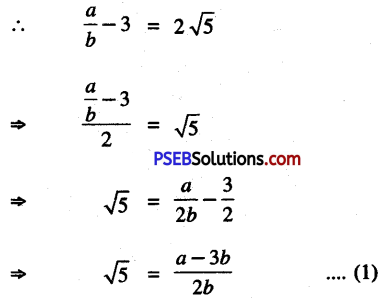# PSEB 10th Class Maths Solutions Chapter 1 Real Numbers Ex 1.3

Punjab State Board PSEB 10th Class Maths Book Solutions Chapter 1 Real Numbers Ex 1.3 Textbook Exercise Questions and Answers.

## PSEB Solutions for Class 10 Maths Chapter 1 Real Numbers Ex 1.3

Question 1.
Prove that √5 is irrational.
Solution:
Let us suppose that √5 is rational so we can find integers r and s where s ≠ 0
such that √5 = $$\frac{r}{s}$$
Suppose r and s have some common factor other than 1, then divide r and s by the common factor to get :
√5 = $$\frac{a}{b}$$ where a and b are coprime and b ≠ 0
b√5 = a
Squaring both sides,
⇒ (b√5)2 = a2
⇒ b2 (√5)2 = a2
⇒ 5b2 = a2 …………..(1)
5 divides a2.
By the theorem, if a prime number ‘p’ divides a2 then ‘p’ divides a where a is positive integer
⇒ 5 divides a …………(2)
So a = 5c for some integer c.
Put the value of a in (1),
5b2 = (5c)2
5b2 = 25c2
b2 = 5c2
or 5c2 = b2
⇒ 5 divides b2
∵ if a prime number ‘p’ divides b2, then p divides b ; where b is positive integer.
⇒ 5 divides b ………… (3)
From (2) and (3), a and b have at least 5 as common factor.
But this contradicts the fact that a and b are coprime i.e. no common factor other than 1.
∴ our supposition that √5 is rational wrong.
Hence √5 is irrational.Question 2.
Prove that 3 + 2 √5 is irrational.
Solution:
Let us suppose that 3 + 2√5 is rational.
∴ we can find Co-Prime a and b, where a and b are integers and b ≠ 0
such that 3 + 2√5 = $$\frac{a}{b}$$Since a and b both are integers,

∴ $$\frac{a-3 b}{2 b}=\frac{\text { (integer) }-3 \text { integer }}{2 \times \text { integer }}$$ = rational number

Hence from (1), √5 is rational.
But this contradicts the fact that √5 is irrational.
∴ our supposition is wrong.
Hence 3 + 2√5 is irrational.Question 3.
Prove that the following are irrationals :
(i) $$\frac{1}{\sqrt{2}}$$
(ii) 7√5
(iii) 6 + √2
Solution:
(i) Given that $$\frac{1}{\sqrt{2}}=\frac{1}{\sqrt{2}} \times \frac{\sqrt{2}}{\sqrt{2}}$$

$$\frac{1}{\sqrt{2}}=\frac{1}{\sqrt{2}} \times \frac{\sqrt{2}}{\sqrt{2}}$$

Let us suppose that $$\frac{\sqrt{2}}{2}$$ is rational
∴ we can find co-prime integers a, b and b ≠ 0.

⇒ $$\frac{\sqrt{2}}{2}=\frac{a}{b}$$

⇒ √2 = $$\frac{2 a}{b}$$ …………….(1)
because division of two integers is a rational number.
So $$\frac{2 a}{b}$$ = rational number

∴ from (1), √2 is also a rational number, which contradicts the fact that J2 is irrational.
∴ our supposition is wrong.

Hence $$\frac{1}{\sqrt{2}}$$ is irrational.(ii) Given that 7√5
Let us suppose that 7^5 is rational
∴ we can find coprime integers a and b where b ≠ 0
such that 7√5 = $$\frac{a}{b}$$
⇒ 7b√5 = a
⇒ √5 = $$\frac{a}{7 b}$$ ……………..(1)
Since a, 7 and b are integers, of two integers is a rational number.
i.e., $$\frac{a}{7 b}$$ = rational number
∴ from (1)
√5 = rational number
which contradicts the fact that √5 is irrational number.
∴ Our supposition is wrong.
Hence 7√5 is irrational.(iii) Given that 6 + √2
Let us suppose that 6 + √2 is rational
∴ we can find coprime integers a and b where b ≠ 0
such that 6 + √2 = $$\frac{a}{b}$$

∴ $$\frac{a}{b}$$ – 6 = √2

or √2 = $$\frac{a-6 b}{b}$$ ………………(1)
Since a and b are integers

∴ $$\frac{a-6 b}{b}=\frac{\text { integer }-6 \times \text { integer }}{\text { integer } \neq 0}$$

[∵ Subtraction of integers is also an integer]

= $$\frac{\text { integer }}{\text { integer } \neq 0}$$ = rational number

[∵ Division of two integers is a rational number]

⇒ $$\frac{a-6 b}{b}$$ = rational number

so from (1), √2 = rational number
which contradicts the fact that √2 is irrational number
∴ Our Supposition is wrong.
Hence 6 + √2 is irrational.